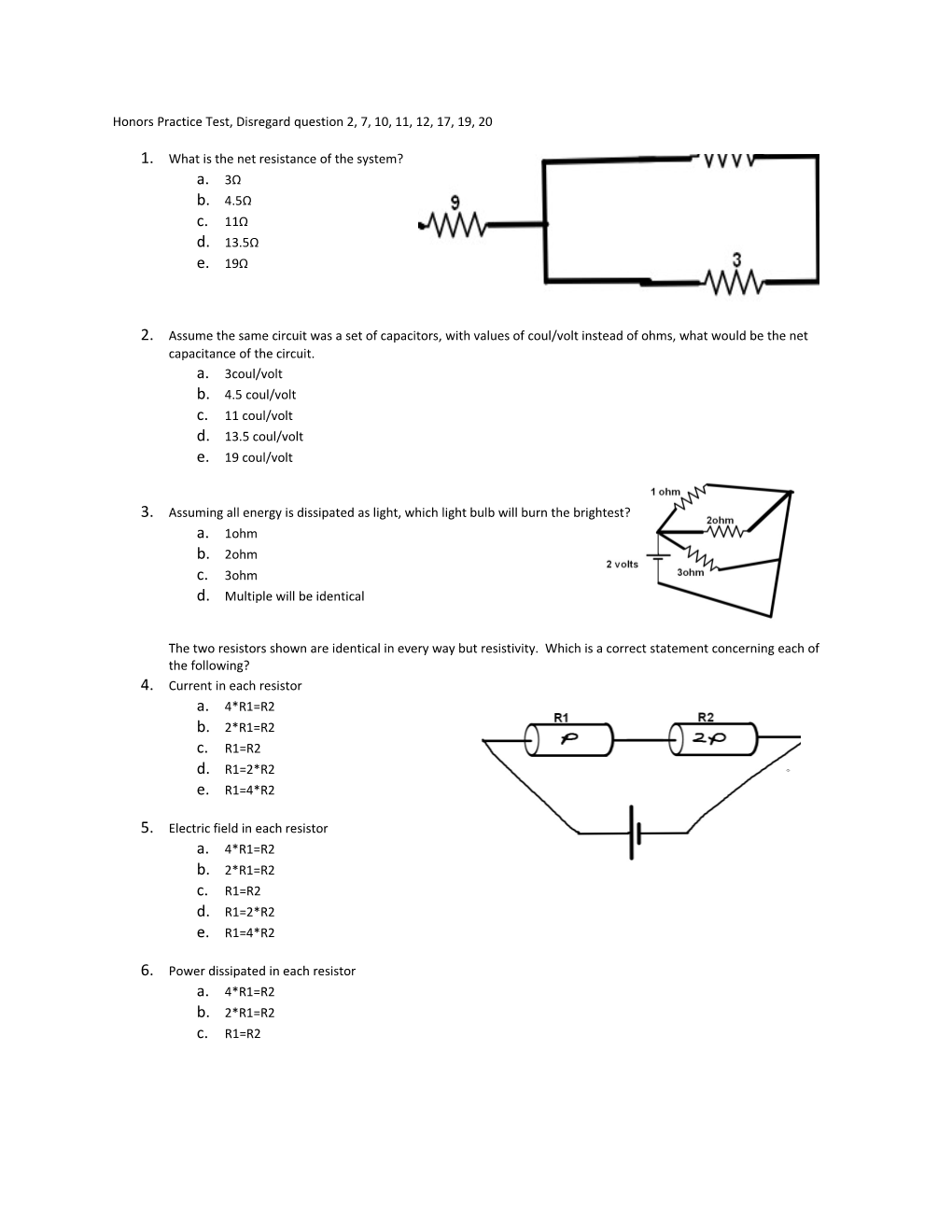# Honors Practice Test, Disregard Question 2, 7, 10, 11, 12, 17, 19, 20Honors Practice Test, Disregard question 2, 7, 10, 11, 12, 17, 19, 20

1. What is the net resistance of the system?
2. 3Ω
3. 4.5Ω
4. 11Ω
5. 13.5Ω
6. 19Ω
1. Assume the same circuit was a set of capacitors, with values of coul/volt instead of ohms, what would be the net capacitance of the circuit.
2. 3coul/volt
3. 4.5 coul/volt
4. 11 coul/volt
5. 13.5 coul/volt
6. 19 coul/volt
1. Assuming all energy is dissipated as light, which light bulb will burn the brightest?
2. 1ohm
3. 2ohm
4. 3ohm
5. Multiple will be identical

The two resistors shown are identical in every way but resistivity. Which is a correct statement concerning each of the following?

1. Current in each resistor
2. 4*R1=R2
3. 2*R1=R2
4. R1=R2
5. R1=2*R2
6. R1=4*R2
1. Electric field in each resistor
2. 4*R1=R2
3. 2*R1=R2
4. R1=R2
5. R1=2*R2
6. R1=4*R2
1. Power dissipated in each resistor
2. 4*R1=R2
3. 2*R1=R2
4.R1=R2
5. R1=2*R2
6. R1=4*R2
1. Which circuit set will discharge the “slowest”, considering time constants? Assume all capacitors are charged to the same voltage and the resistors are identical.

The following applies to the next two questions

1. In the circuit shown, all the resistors shown have a resistance R; these are labeled R1, R2, and R3. Assume R>r. If the switch is closed, the terminal voltage of the battery will ______and the brightness of bulb R2 will ______
2. Decrease, Decrease
3. Decrease, Increase
4. Decrease, Stay the same
5. Increase, Decrease
6. Increase, Increase
1. Which of the following is true as the switch is closed?
2. Light bulb R3 will become dimmer
3. R1 will slowly increase in brightness to a maximum after the switch is closed
4. The Emf of the battery increases
5. The power output of the battery increases
6. None of the above

The two circuits shown are identical in every way but the gap distance between the capacitors, CA and CB. They are allowed to fully charge with identical batteries.

1. Compare the capacitance.
2. CA<CB
3. CA=Cb
4. CA>Cb
5. Impossible to determine
1. Compare the charge stored on each capacitor
2. CA<CB
3. CA=Cb
4. CA>Cb
5. Impossible to determine
1. Compare the electric field between the capacitor plates.
2. CA<CB
3. CA=Cb
4. CA>Cb
5. Impossible to determine
1. Compare the voltage on each capacitors
2. CA<CB
3. CA=Cb
4. CA>Cb
5. Impossible to determine

Considering two resistors R1 and R2 with the relative dimensions shown (the width of each resistor is different and the lengths are the same), solve the following. Assume the resistors are the same material and length. Which statement is true regarding their

1. Resistance
2. R1<R2
3. R1=R2
4. R1>R2
5. Cannot be determined from information given
1. Electric field
2. R1<R2
3. R1=R2
4. R1>R2
5. Cannot be determined from information given
1. Current
2. R1<R2
3. R1=R2
4. R1>R2
5. Cannot be determined from information given
1. Drift velocity
2. R1<R2
3. R1=R2
4. R1>R2
5. Cannot be determined from information given
1. Considering the glimpse of the circuit shown (the rest of the circuit is shielded from view), what is the change in voltage from a to b?
2. -40
3. -24
4. 0
5. 24
6. 40
1. A Dielectric is inserted halfway into a capacitor with capacitance C. If the dielectric has a constant of 8, what is the new capacitance, in terms of C.
2. 1.5C
3. 4.5C
4. 5C
5. 8C
6. 9C
1. After 30seconds Voltage on the capacitor will fall in which range
2. 0-5 volts
3. 5-10 volts
4. 10-15volts
5. 15-20volts
6. None of the above